• 目前相关方面的研究很少，本文以某一型号N沟道增强型MOS场效应管组成的分压-自偏压共源放大电路为例，进行静态、动态和温度特性分析，并且与理论计算结果对比，得出分压-自偏压共源放大电路的Multisim电路仿真分析...
• 自偏压共源放大电路的Multisim仿真研究pdf,自偏压共源放大电路的Multisim仿真研究
• 目前相关方面的研究很少，本文以某一型号N沟道增强型MOS场效应管组成的分压-自偏压共源放大电路为例，进行静态、动态和温度特性分析，并且与理论计算结果对比，得出分压-自偏压共源放大电路的Multisim电路仿真分析...
• 针对场效应管电路分析中不同元件性能参数不同而导致一些理论计算复杂、繁琐，并且难于理解的情况，通过对N沟道增强型MOS场效应管组成的分压-自偏压共源放大电路的理论研究，利用Multisim仿真软件对电路实际工作情况...
• 在Multisim软件中绘制的分压-自偏压共源放大电路仿真电路，附带电路截图加输入输出波形，在由 N 沟道增强型 MOS 场效应管组成的分压-自 偏压共源放大电路中 ,由于所选用场效应管的性能参数 不同 ,在理论计算中要考虑...Multisim
• 在Multisim软件中绘制的分压-自偏压共源放大电路仿真电路，附带电路截图加输入输出波形。
• UGSQU_{GSQ}UGSQ​对共源放大电路电压放大倍数的影响 电路搭建（采用增强型MOSFET）恒流区iD=IDO(UGSUGS(th)−1)2i_D=I_{DO}(\frac{U_{GS}}{U_{GS(th)}}-1)^2iD​=IDO​(UGS(th)​UGS​​−1)2 仿真分析1 采用参数...
$U_{GSQ}$对共源放大电路电压放大倍数的影响
电路搭建（采用增强型MOSFET）恒流区$i_D=I_{DO}(\frac{U_{GS}}{U_{GS(th)}}-1)^2$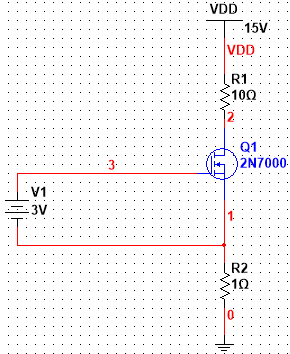仿真分析1
采用参数扫描分析方法，设置直流电源V1为被扫描电源，节点1为输出，又源极电阻为1$\Omega$，因此节点1的输出可视为漏（源）极电流。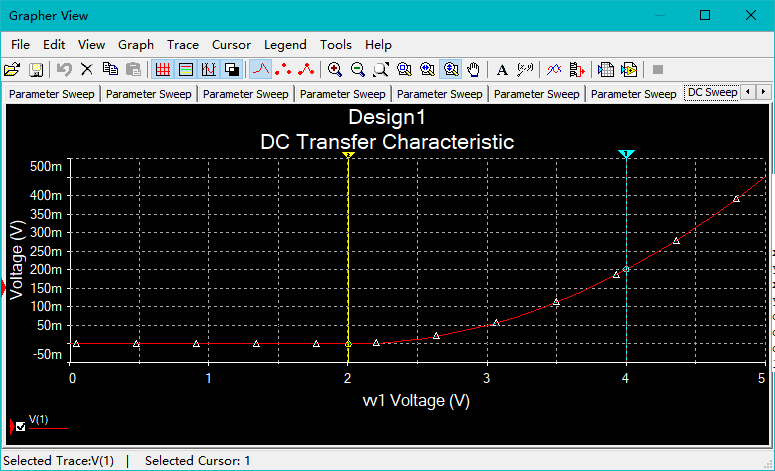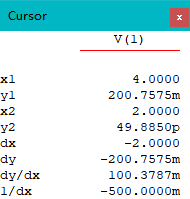发现，该增强型MOSFET的开启电压$U_{GS(th)}$=2V，$I_{DO}\approx200mA$ （$I_{DO}$是当$U_{GS}$=2$U_{GS(th)}$时的漏极电流）
仿真分析2
在$R_{g2}$分别为6M$\Omega$和6.1M$\Omega$时，采用电压表和示波器测$U_{GSQ}$、$U_{DSQ}$、$U_o$。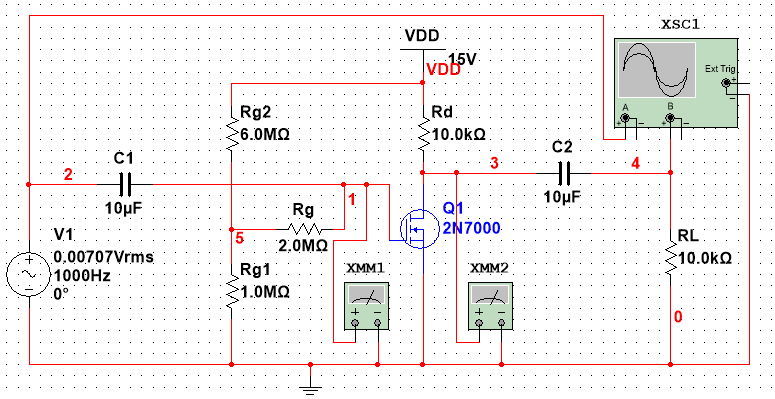$R_{g2}$=$6M\Omega$时，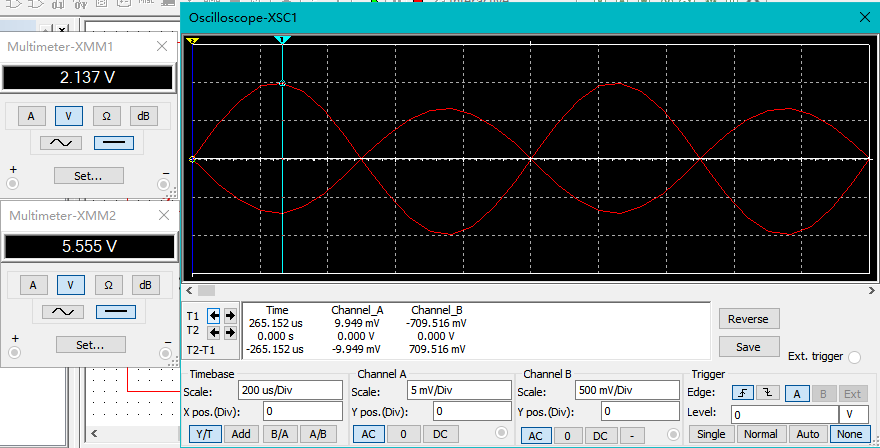$U_{GSQ}$=2.137V，$U_{DSQ}$=5.555V $\Rightarrow$ $I_{DQ} \approx 0.938uA$
$U_o\approx$ 710mV
$R_{g2}$=$6.1M\Omega$时，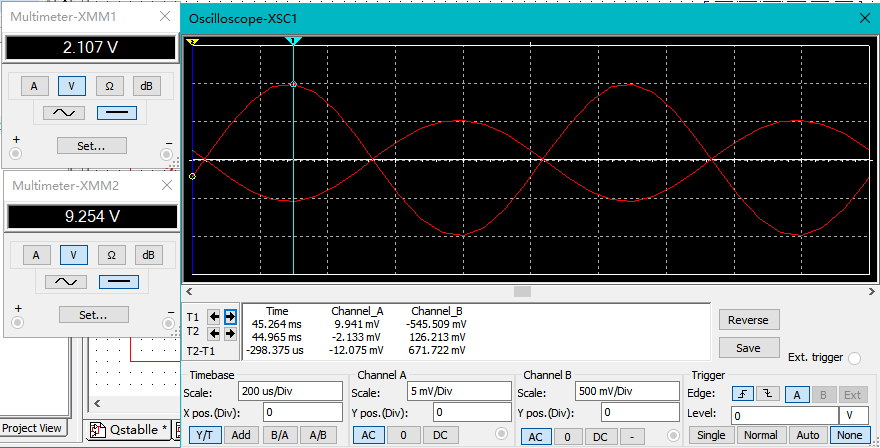$U_{GSQ}$=2.107V，$U_{DSQ}$=9.254V $\Rightarrow$ $I_{DQ} \approx 0.572uA$
$U_o\approx$ 546mV

输入电压峰值/mV
$R_{g2}/M\Omega$
$U_{GSQ}$/V
$U_{DSQ}$/V
$I_{DQ}$/uA
$U_o/mV$
电压放大倍数Av

10
6.0
2.137
5.555
0.938
710
-71.0

10
6.1
2.107
9.254
0.572
546
-54.6

随着$R_{g2}$增大，$U_{GSQ}$减小， $I_{DQ}$减小，电压放大倍数减小
$\Rightarrow$ 调整偏置电阻，使$U_{GSQ}$增大， $I_{DQ}$增大，从而提高电压放大能力。当然要保证管子工作在恒流区，不失真，即$U_{GS}>U_{GS(th)}$，$U_{GD}
理论计算
$g_m=\frac{\partial i_d}{\partial u_{GS}}$
$\Rightarrow g_m=\frac{2I_{DO} }{U_{GS(th)}}(\frac{U_{GS}}{{U_{GS(th)}}}-1)$
$\Rightarrow$ $g_{m1}=0.0137S; g_{m2}=0.0137S$
$\Rightarrow A_v=-g_mR_d||R_L$
$\Rightarrow A_{v1}=-68.5;A_{v2}=-53.5$
理论计算与实验结果比较

输入电压峰值/mV
$R_{g2}/M\Omega$
$U_{GSQ}$/V
$U_{DSQ}$/V
$I_{DQ}$/uA
$U_o/mV$
电压放大倍数Av（实验值）
电压放大倍数Av（理论计算）

10
6.0
2.137
5.555
0.938
710
-71.0
-68.5

10
6.1
2.107
9.254
0.572
546
-54.6
-53.5

说明，公式的近似效果甚好，同时也说明了仿真对电路调试的实际指导意义


展开全文• NMOS管共源放大实验电路multisim源文件，multisim10及以上版本可以正常打开仿真，是教材上的电路，可以直接仿真，方便大家学习。multisim
• 本文简述漏极电流Id与漏源电压和栅源电压的关系及小信号模型分析方法。
• MOSFET理解与应用：Lec 12—一篇文章搞定共源放大电路 https://baijiahao.baidu.com/s?id=1616701539915827630&wfr=spider&for=pc 为了便于保存和引用才进行转载学习 本期内容： 共源放大电路的偏置...
转载文章来自：

MOSFET理解与应用：Lec 12—一篇文章搞定共源级放大电路
https://baijiahao.baidu.com/s?id=1616701539915827630&wfr=spider&for=pc

为了便于保存和引用才进行转载学习

本期内容：

共源级放大电路的偏置设计

共源级放大电路的小信号分析

——————————————————————————————————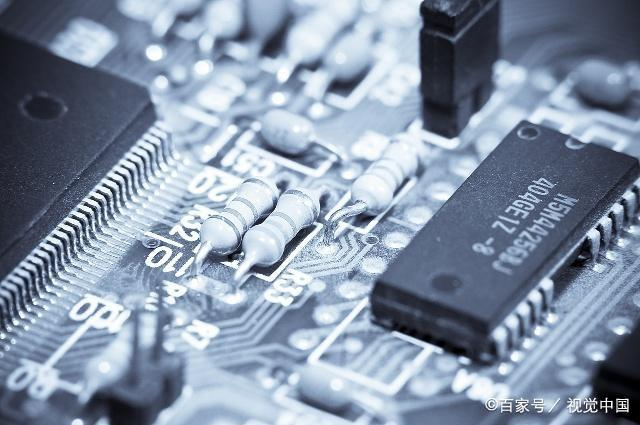1、共源级放大器的偏置电路设计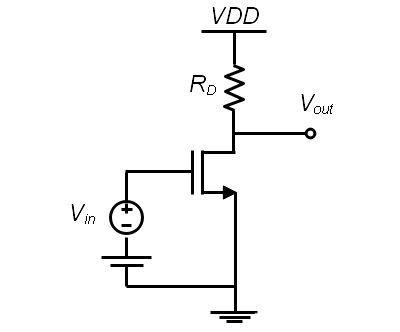Fig. 1

一个合适的偏置电路设计是放大器工作的前提，偏置电路需要使MOSFET工作在饱和区。如图Fig. 1，这是一个前几期讲过的MOSFET放大器的最基本电路。

① 电阻分压偏置电路

Fig. 2是一个最简单常见的偏置电路的设计，对比Fig. 1有很多不用，首先栅极没有了偏置直流电源，多了好几个电阻，多了一个电容，这些器件都有什么用呢？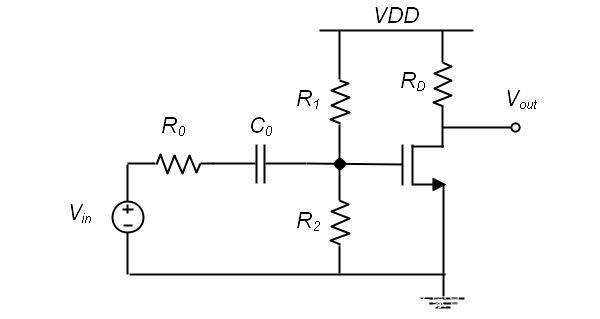Fig. 2

Fig. 1中的供电设计是复杂的，需要两种电源才能使放大器工作，这个是我们不希望的，所以Fig. 2中利用电阻的分压电路，使VDD分一部分电压给栅极提供偏置电压，大小为VDD*R2/(R1+R2)。这样整个系统就可以只用一个供电单元来提供电压，简化了设计。所以R1，R2的作用是分压，为MOSFET提供栅极开启电压。Fig. 2 中的R0为小信号源的内阻，这个内阻在实际电路设计中是必须要考虑的，比如要放大的信号时麦克风信号，那R0就表示麦克风的内阻。Fig. 2 中的C0为一个隔直电容，作用是防止前级直流信号对后级偏置电压的影响。只有交流信号能够通过后级电路进行放大。但是电容对于MOSFET器件来说是一个非常大的器件，增加一个电容会占用芯片很大一块位置，所以尽量避免使用电容，如图Fig. 3有两级放大电路，如果前一级的输出Vx的直流部分正好可以作为后级的直流偏置，则不需要加电容和分压电阻。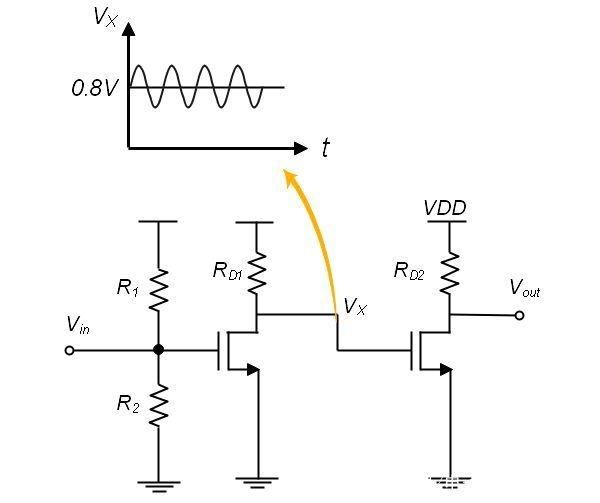Fig. 3

选择合适的R0，R1，RD才能使MOSFET工作在饱和区，使其具有放大作用。下期讲介绍一个实际的例子来说明这些电阻应该如何选取，大概的量级是多少。

② 自偏置电路

Fig. 4是一个自偏置电路的设计，此时，通过RF的电流为零，RF两边的电压相等，MOSFET的漏极电压和栅极电压相等，都等于VDD-I_D * R_D。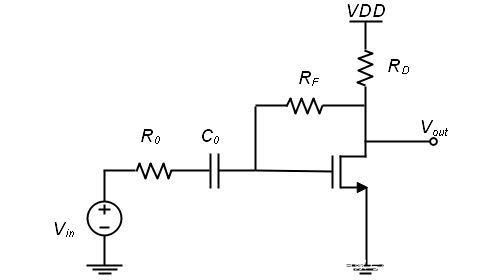Fig. 4

自偏置电路的好处是它对MOSFET的截止电压的敏感性弱，因为工艺的公差会导致两片晶圆片的阈值电压不可能完全相等，比如晶圆片A的阈值电压为V_TH，A，晶圆片B的阈值电压为V_TH，B，如下图Fig. 5，如果用电阻分压方法来提供栅极的偏置电压，则相同的V_GS在两个晶圆片上得到的漏极电流是不同的，这就会导致两片晶圆片做出来的放大器的放大倍数不相同。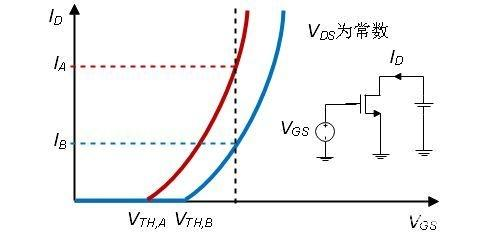Fig. 5

但如果用Fig. 4的自偏压电路，V_TH上升会导致I_D下降，I_D下降会导致V_{GS}上升，V_{GS}上升又会导致I_D上升，这个电路有个自反馈的过程在，所以他对V_TH的变化敏感性不高，在一定程度上晶圆片的公差不过过大的影响放大器的性能。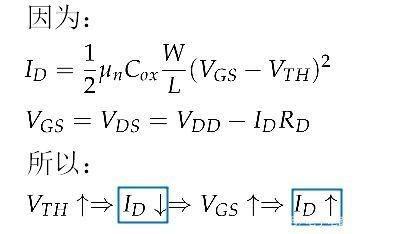共源级放大电路的偏置部分就介绍这两种，其他种类的偏置电路大同小异。

2、共源级放大电路的小信号分析

放大电路的小信号分析主要考虑三个参数，放大倍数、输入阻抗和输出阻抗，这三个参数直接影响实际电路中的小信号放大倍数。我们以电阻分压偏置电路Fig. 2为例进行计算这三个参数。这里主要运用MOSFET的小信号电路模型和输入阻抗和输出阻抗的概念。不清楚的请查看。MOSFET理解与应用：Lec 9—NMOS、PMOS小信号电路模型总结、MOSFET理解与应用：Lec 10—输入阻抗和输出阻抗的概念。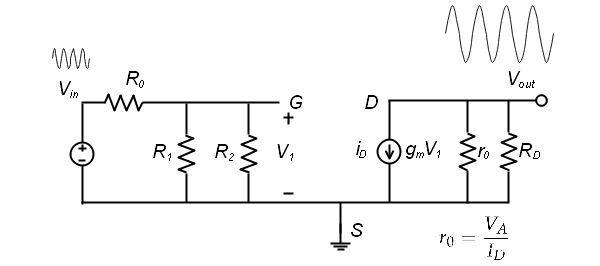Fig. 6

如图Fig. 6，为电阻分压偏置电路的小信号电路模型，这里只是将Lec 9 中的模型替换了MOSFET器件，是不是超级简单。通过这个电路图可以求出：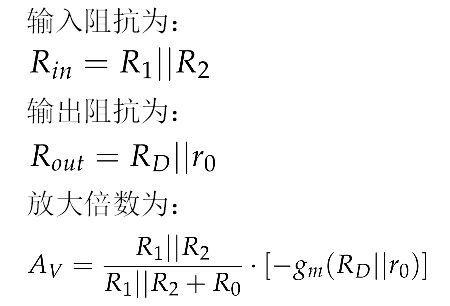从这个式子可以看出要使放大倍数提高，需要R1||R2远大于R0。这也是偏置电路设计时需要注意的一点。

总结一下：偏置电路是设计是为了放大器能够正常工作，使MOSFET工作在饱和区，同时引入的器件如电阻也会影响MOSFET的放大倍数。然后利用小信号模型求出具体的放大倍数，输入输出阻抗这三个变量。

展开全文• 1.要求： a.增益10db Av= 20log(Vout/Vin) Vout/Vin = 3; b.... ...2.管子种类不一样，IDSS不一样，2SK184GR IDSS范围：2.6ma~6.5...IDSS即可，此处为了安全起见，选择ID=1Ma.Id决定了传导率和噪声，所以设计电路从ID...
1.要求：

a.增益10db  Av= 20log(Vout/Vin)     Vout/Vin  = 3;

b.峰峰值：3Vp-p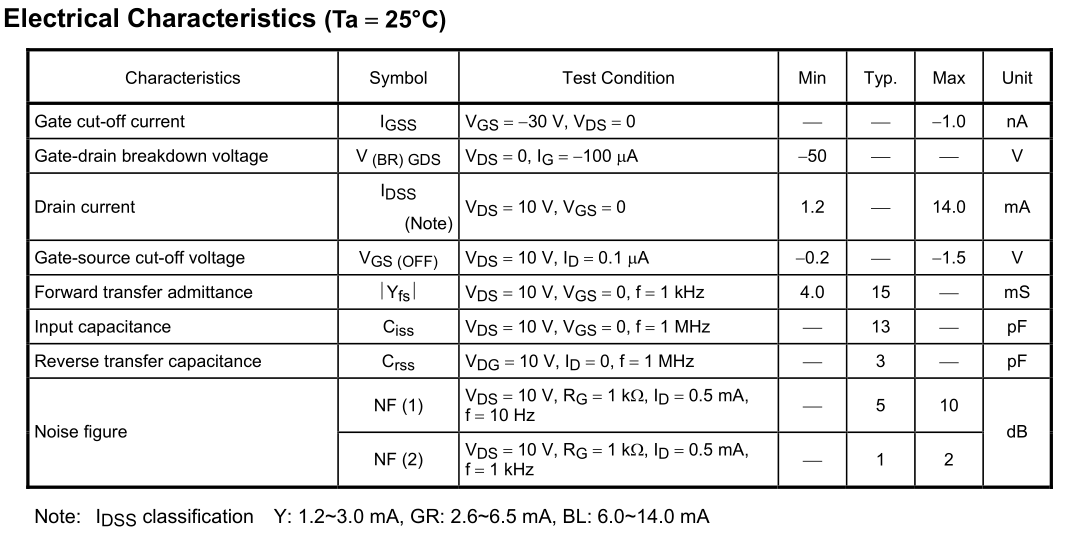2.管子种类不一样，IDSS不一样，2SK184GR  IDSS范围：2.6ma~6.5Ma。

取Id值，设计RD\RS阻值，取值条件只需满足<IDSS即可，此处为了安全起见，选择ID=1Ma.Id决定了传导率和噪声，所以设计电路从ID开始。
RS=VS/I=2V/1Ma= 2K，   VS选择在1V以上，避免温度变化带来的偏移。

RD=Av*Rs= 3*2K= 6K。

功耗计算：P=(15V-1ma*2k-1ma*6k)*I=7V*1ma = 7mW.   小于2SK184GR  PD 200mW。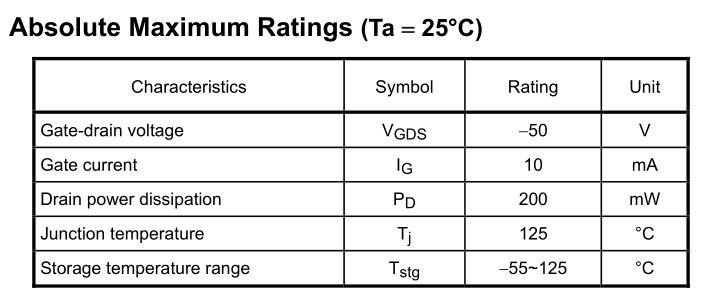栅极偏压电路设计
2SK184GR 传输特性曲线：IDSS在1MA左右时，根据上图VGS范围在-0.1~0.4之间，取中间值-0.25V，然后得到VG=2v-0.25V=1.75v,R1电阻压降1.75V，R2电阻压降13.25V。因JFET 输入电阻非常大，G\S间电流几乎为0.

R2 =20 K，R1=150K。

Pspice 采用BC264A做仿真，仿真原理图如下：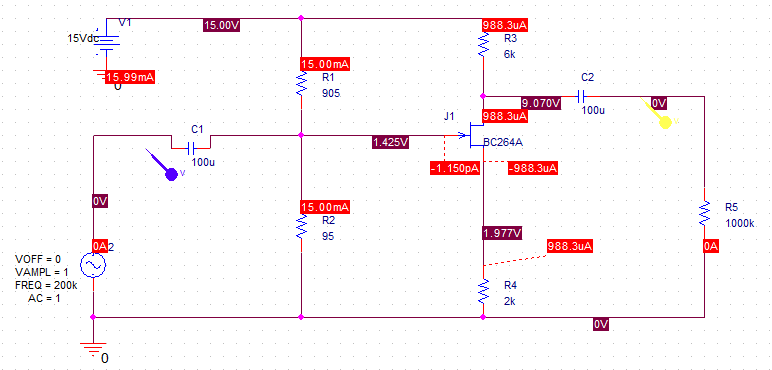Q:

为什么放大倍数只有2.5倍左右？排查发现S级电压增量和Vin输入电压不一样实际没有2Vp-p.红色为输入信号VIN，绿色为Vs增量。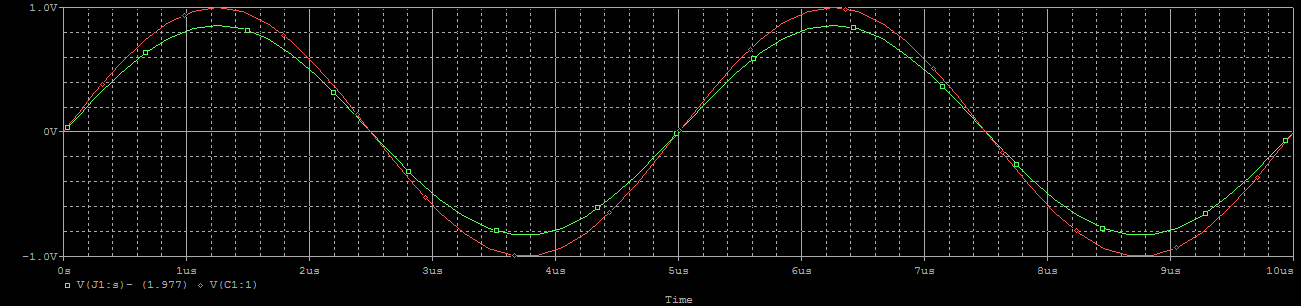展开全文• 低频响应(1) 共射放大电路(2) 共源放大电路2. 高频响应(1) 共射放大电路(2) 共基放大电路(3) 共集放大电路(4) 共源放大电路(5) 共栅放大电路(6) 共漏放大电路(7) 多级放大电路 0. 单时间常数RC电路的频率响应 (1) ...
目录0. 单时间常数RC电路的频率响应(1) RC高通电路(2) RC低通电路1. 低频响应(1) 共射放大电路(2) 共源放大电路2. 高频响应(1) 共射放大电路(2) 共基放大电路(3) 共集放大电路(4) 共源放大电路(5) 共栅放大电路(6) 共漏放大电路(7) 多级放大电路
0. 单时间常数RC电路的频率响应
(1) RC高通电路

对应的为低频响应。

电路图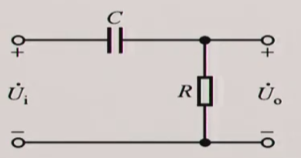增益
$\dot{A}_{vL} = \frac{\dot{V}_o}{\dot{V}_i} = \frac{1}{1 - j\frac{f_L}{f}}$
② 相频响应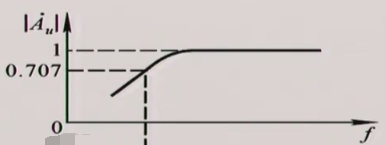$|\dot{A}_{vL}| = \frac{1}{\sqrt{1+(\frac{f_L}{f})^2}}$
曲线分析

当
当
当

③  幅频响应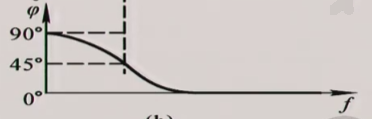$φ_L = arctan(\frac{f_{L}}{f})$
曲线分析

当
当
当

(2) RC低通电路① 传输特性
$\dot{A}_u = \frac{\dot{U}_o}{\dot{U}_i} = \frac{1}{1+\frac{1}{j\omega RC}}$

下限截止频率$f_L = \frac{1}{2\pi RC}$

② 幅频特性
$|\dot{A}_u| = \frac{1}{\sqrt{1+(\frac{f}{f_H})^2}}$
曲线分析

当 $f 时，
当 $f=f_H$ 时，
当 $f>f_H$ 时，

③ 相频响应
$\phi_h= -arctan(\frac{f}{f_H})$
曲线分析

当 $f 时，
当 $f=f_H$ 时，
当 $f>f_H$ 时，

1. 低频响应
(1) 共射放大电路
(2) 共源放大电路
2. 高频响应
(1) 共射放大电路
分析步骤

根据BJT混合$\pi$形高频小信号模型，画等效电路
求密勒电容，再次等效，把电容单向化
根据电容间的关系，再次简化，
利用戴维宁等效电路进行简化
求解高频响应和上限频率。

(2) 共基放大电路
(3) 共集放大电路
(4) 共源放大电路
(5) 共栅放大电路
(6) 共漏放大电路
(7) 多级放大电路


展开全文• 带源极电阻的共源放大电路能否解决因mosfet参数的分散性(由生产工艺造成)而引起的静态工作点不稳定的问题？
• 　基于结型场效应晶体管或“ JFET”（本教程中为N沟道FET）甚至金属氧化物硅FET或“ MOSFET”为基础的放大电路的设计与双极晶体管电路的原理完全相同用于上一教程中介绍的A类放大器电路。　首先，需要找到合适的静态...
• MOSFET基本共源放大电路 基本共源放大电路的组成 基本共源放大电路的工作原理 放大电路的习惯画法和主要分析法 图解分析法 用图解方法确定静态工作点Q 动态工作情况的图解分析 图解分析法的适用范围 小信号...
• 写在前面：本博文是华南理工大学电子与信息学院...基本共源放大电路3.2.基本共漏放大电路 1.场效应管介绍 2.场效应管静态工作点的设置以及求解 首先，对于场效应管来说，我们静态工作点Q的指标包括：IDQ,UGSQ,U...场效应管
• 在上一章中，我们讲述了最基本的带电阻的共源放大电路，这也是很多放大电路的基础，但是，对于带电阻的共源放大电路而言存在不少缺点，主要有： 电阻制作工艺不能制造出一个具有绝对精确阻值的电阻 电阻的阻值并不...
• 基本共源放大电路 根据场效应管工作在恒流区的条件，在栅极与源极之间增加正向电压，在漏极与源极之间增加合适极性与大小的电压。 1.静态工作点的确定 二.自给偏压电路 上图中的管子是结型N沟道场效应管，即使在...
• 其在输入回路中串接一取样电阻R，输入信号调整在放大电路用晶体管毫对地的交流电压VS与Vi，这样求得两端的电压为VR=VS-Vi，流过电阻R的电流实际就是放大电路的输入电流Ii。 　根据输入电阻的定
• 和半导体三极管一样，场效应管的电路也有三种接法，即共源极电路、共漏极...　共源极基本放大电路的主要参数，可由以下各式确定:　输入电阻Ri=RG//RGS，因RGS》RG，故 Ri≈RG　输出电阻R。=rDS//RL，因rDS》RL，故
• 功率放大电路一. 功率放大电路的三种工作状态1.甲类工作状态导通角为360度，ICQ大，管耗大，效率低。 2.乙类工作状态ICQ≈0， 导通角为180度，效率高，失真大。3.甲乙类工作状态 导通角为180o~360o，效率较高，失真...
• 共射-共基放大电路 共集-共集放大电路 共源-共集放大电路
• 和半导体三极管一样，场效应管的电路也有三种接法即共源电路、共漏极电路和共栅极电路。本文简述场效应管电路的这三种接法。
• 学习了晶体管和场效应管的单级放大电路，但是我们会发现很多时候单级放大电路不能同时满足多个条件时，比如：此处放大倍数很大，单级放大电路明显不能满足要求。此时就需要耦合连接多个放大电路。：直接耦合T1的静态......